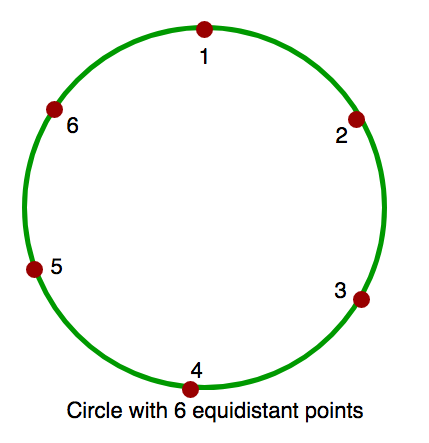# Count of obtuse angles in a circle with ‘k’ equidistant points between 2 given points

A circle is given with k equidistant points on its circumference. 2 points A and B are given in the circle. Find the count of all obtuse angles (angles larger than 90 degree) formed from /_ACB, where C can be any point in circle other than A or B.
Note :
A and B are not equal.
A < B.
Points are between 1 and K(both inclusive).Examples :

```Input : K = 6, A = 1, B = 3.
Output : 1
Explanation : In the circle with 6
equidistant points, when C = 2 i.e.
/_123, we get obtuse angle.

Input : K = 6, A = 1, B = 4.
Output : 0
Explanation : In this circle, there
is no such C that form an obtuse angle.
```

## Recommended: Please try your approach on {IDE} first, before moving on to the solution.

It can be observed that if A and B have equal elements in between them, there can’t be any C such that ACB is obtuse. Also, the number of possible obtuse angles are the smaller arc between A and B.

Below is the implementation :

## C++

 `// C++ program to count number of obtuse ` `// angles for given two points. ` `#include ` `using` `namespace` `std; ` ` `  `int` `countObtuseAngles(``int` `a, ``int` `b, ``int` `k) ` `{ ` `    ``// There are two arcs connecting a ` `    ``// and b. Let us count points on ` `    ``// both arcs. ` `    ``int` `c1 = (b - a) - 1; ` `    ``int` `c2 = (k - b) + (a - 1); ` ` `  `    ``// Both arcs have same number of ` `    ``// points ` `    ``if` `(c1 == c2) ` `        ``return` `0; ` ` `  `    ``// Points on smaller arc is answer ` `    ``return` `min(c1, c2); ` `} ` ` `  `// Driver code ` `int` `main() ` `{ ` `    ``int` `k = 6, a = 1, b = 3; ` `    ``cout << countObtuseAngles(a, b, k); ` `    ``return` `0; ` `} `

## Java

 `// Java program to count number of obtuse ` `// angles for given two points ` `class` `GFG { ` ` `  `    ``static` `int` `countObtuseAngles(``int` `a, ` `                                 ``int` `b, ``int` `k) ` `    ``{ ` ` `  `        ``// There are two arcs connecting a ` `        ``// and b. Let us count points on ` `        ``// both arcs. ` `        ``int` `c1 = (b - a) - ``1``; ` `        ``int` `c2 = (k - b) + (a - ``1``); ` ` `  `        ``// Both arcs have same number of ` `        ``// points ` `        ``if` `(c1 == c2) ` `            ``return` `0``; ` ` `  `        ``// Points on smaller arc is answer ` `        ``return` `min(c1, c2); ` `    ``} ` ` `  `    ``// Driver Program to test above function ` `    ``public` `static` `void` `main(String arg[]) ` `    ``{ ` ` `  `        ``int` `k = ``6``, a = ``1``, b = ``3``; ` `        ``System.out.print(countObtuseAngles(a, b, k)); ` `    ``} ` `} ` ` `  `// This code is contributed by Anant Agarwal. `

## Python

 `# C++ program to count number of obtuse ` `# angles for given two points. ` ` `  `def` `countObtuseAngles( a, b, k): ` `    ``# There are two arcs connecting a  ` `    ``# and b. Let us count points on ` `    ``# both arcs. ` `    ``c1 ``=` `(b ``-` `a) ``-` `1` `    ``c2 ``=` `(k ``-` `b) ``+` `(a ``-` `1``) ` `  `  `    ``# Both arcs have same number of ` `    ``# points ` `    ``if` `(c1 ``=``=` `c2): ` `       ``return` `0` `      `  `    ``# Points on smaller arc is answer ` `    ``return` `min``(c1, c2) ` `  `  `# Driver code ` `k, a, b ``=` `6``, ``1``, ``3` `print` `countObtuseAngles(a, b, k) ` ` `  `# This code is contributed by Sachin Bisht `

## C#

 `// C# program to count number of obtuse ` `// angles for given two points ` `using` `System; ` ` `  `class` `GFG { ` ` `  `    ``static` `int` `countObtuseAngles(``int` `a, ` `                           ``int` `b, ``int` `k) ` `    ``{ ` ` `  `        ``// There are two arcs connecting ` `        ``// a and b. Let us count points ` `        ``// on both arcs. ` `        ``int` `c1 = (b - a) - 1; ` `        ``int` `c2 = (k - b) + (a - 1); ` ` `  `        ``// Both arcs have same number ` `        ``// of points ` `        ``if` `(c1 == c2) ` `            ``return` `0; ` ` `  `        ``// Points on smaller arc is  ` `        ``// answer ` `        ``return` `Math.Min(c1, c2); ` `    ``} ` ` `  `    ``// Driver Program to test above ` `    ``// function ` `    ``public` `static` `void` `Main() ` `    ``{ ` ` `  `        ``int` `k = 6, a = 1, b = 3; ` `         `  `        ``Console.WriteLine( ` `           ``countObtuseAngles(a, b, k)); ` `    ``} ` `} ` ` `  `// This code is contributed by vt_m. `

## PHP

 ` `

Output :

`1`

This article is contributed by Rohit Thapliyal. If you like GeeksforGeeks and would like to contribute, you can also write an article using contribute.geeksforgeeks.org or mail your article to contribute@geeksforgeeks.org. See your article appearing on the GeeksforGeeks main page and help other Geeks.

Attention reader! Don’t stop learning now. Get hold of all the important DSA concepts with the DSA Self Paced Course at a student-friendly price and become industry ready.

My Personal Notes arrow_drop_up

Improved By : vt_m, jit_t

Article Tags :
Practice Tags :

Be the First to upvote.

Please write to us at contribute@geeksforgeeks.org to report any issue with the above content.